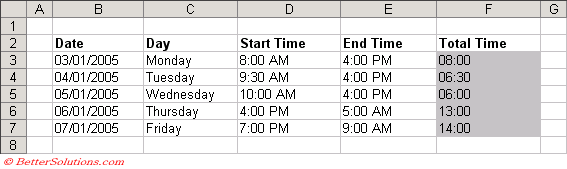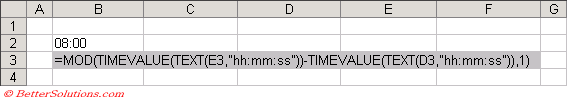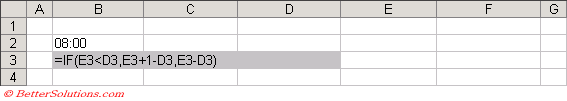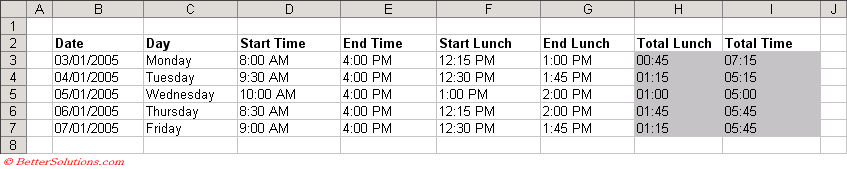### Time Sheet

This example illustrates how to create a simple worksheet to keep track of hours worked during a week.
There are several possible formulas which can be used to calculate the total time.It is very important to format the cells correctly especially when they contain dates and time.
The formats used in the above table are as follows:
Column "D" - h:mm AM/PM;
Column "E" - h:mm AM/PM;
Column "F" - hh:mm;

#### Formula 1

This formula looks quite complicated but is actually very simple and only contains 2 cell references.The advantage of the TIMEVALUE() function is that the date is ignored.

#### Formula 2

This formula is a bit simpler but it contains 6 cell references, meaning there is more room for mistakes.#### Excluding Lunch

You can calculate the total time for lunch using exactly the same formulas as above.
Just change the cell references to refer to the start lunch and end lunch times.
To obtain the total time "actually worked" you can subtract the total lunch time away from the total time.